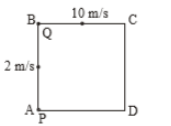A stone falls freely from rest from a height h and it travels a distance $\frac{9h}{25}$ in the last second. The value of h is:

1. 145 m

2. 100 m

3. 122.5 m

4. 200 ms

Concept Questions :-

Uniformly accelerated motion
High Yielding Test Series + Question Bank - NEET 2020

Difficulty Level:

A point moves in a straight line under the retardation a${\mathrm{v}}^{2}$. If the initial velocity is u, the distance covered in 't' seconds is-

1.  $\mathrm{aut}$

2.

3.

4.

Concept Questions :-

Non-uniform acceleration
High Yielding Test Series + Question Bank - NEET 2020

Difficulty Level:

A particle is thrown upwards from ground. It experiences a constant resistance force which can produce retardation of 2 $m/{s}^{2}$. The ratio of time of ascent to the time of descent is:

(1) 1:1

(2) $\sqrt{\frac{2}{3}}$

(3) $\frac{2}{3}$

(4) $\sqrt{\frac{3}{2}}$

Concept Questions :-

Uniformly accelerated motion
High Yielding Test Series + Question Bank - NEET 2020

Difficulty Level:

Two men P & Q are standing at corners A & B of square ABCD of side 8 m. They start moving along the track with constant speed 2 m/s and 10 m/s respectively. The time when they will meet for the first time, is equal to:(1) 2 sec

(2) 3 sec

(3) 1 sec

(4) 6 sec

Concept Questions :-

Relative motion in 1-D
High Yielding Test Series + Question Bank - NEET 2020

Difficulty Level:

A bullet loses $\frac{1}{20}$ of its velocity passing through a plank. The least number of planks required to stop the bullet is (All planks offers same retardation)

(1) 10

(2) 11

(3) 12

(4) 23

Concept Questions :-

Uniformly accelerated motion
High Yielding Test Series + Question Bank - NEET 2020

Difficulty Level:

A body starts from the origin and moves along the X-axis such that the velocity at any instant is given by $\left(4{t}^{3}-2t\right)$, where t is in sec and velocity in m/s. What is the acceleration of the particle, when it is 2 m from the origin ?

1. 28 m/s2

2. 22 m/s2

3. 12 m/s2

4. 10 m/s2

Concept Questions :-

Non-uniform acceleration
High Yielding Test Series + Question Bank - NEET 2020

Difficulty Level:

The relation between time and distance is $t=\alpha {x}^{2}+\beta x$, where α and β are constants. The retardation is

1. $2\alpha {v}^{3}$

2. $2\beta {v}^{3}$

3. $2\alpha \beta {v}^{3}$

4. $2{\beta }^{2}{v}^{3}$

Concept Questions :-

Instantaneous speed and instantaneous velocity
High Yielding Test Series + Question Bank - NEET 2020

Difficulty Level:

A point moves with uniform acceleration and v1, v2 and v3 denote the average velocities in the three successive intervals of time t1, t2 and t3. Which of the following relations is correct ?

(1) $\left({v}_{1}-{v}_{2}\right):\left({v}_{2}-{v}_{3}\right)=\left({t}_{1}-{t}_{2}\right):\left({t}_{2}+{t}_{3}\right)$

(2) $\left({v}_{1}-{v}_{2}\right):\left({v}_{2}-{v}_{3}\right)=\left({t}_{1}+{t}_{2}\right):\left({t}_{2}+{t}_{3}\right)$

(3) $\left({v}_{1}-{v}_{2}\right):\left({v}_{2}-{v}_{3}\right)=\left({t}_{1}-{t}_{2}\right):\left({t}_{1}-{t}_{3}\right)$

(4) $\left({v}_{1}-{v}_{2}\right):\left({v}_{2}-{v}_{3}\right)=\left({t}_{1}-{t}_{2}\right):\left({t}_{2}-{t}_{3}\right)$

Concept Questions :-

Uniformly accelerated motion
High Yielding Test Series + Question Bank - NEET 2020

Difficulty Level:

The acceleration of a moving body can be found from

(1) Area under velocity-time graph

(2) Area under distance-time graph

(3) Slope of the velocity-time graph

(4) Slope of distance-time graph

Concept Questions :-

Graphs
High Yielding Test Series + Question Bank - NEET 2020

Difficulty Level:

The initial velocity of a particle is u (at t = 0) and the acceleration f is given by at. Which of the following relation is valid

1. $v=u+a{t}^{2}$

2. $v=u+a\frac{{t}^{2}}{2}$

3. $v=u+at$

4. v = u

Concept Questions :-

Non-uniform acceleration